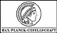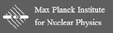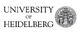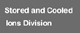Superordinated navigation: MPIK Homepage  |  Home  |  Deutsch  |  Sitemap  |  Search  |  Contact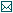Contact

Tel.: +49 6221 516-851
Fax: +49 6221 516-852
Max Planck Institute for Nuclear Physics
P.O. Box 10 39 80
69029 Heidelberg
Max Planck Institute for Nuclear Physics
Saupfercheckweg 1
Building: Gentner lab,
room 134
69117 Heidelberg

# THe-TRAP Project

## Detection method

The stored ion induces image charges on the surfaces of the trap electrodes. The oscillating ion movement creates an image current. For detecting the axial frequency of the ion, a tuned resonant circuit is used, to which a cryogenic amplifier is connected. A sketch of the detection system can be found in Figure 5.1.Fig. 5.1: A sketch of the ion detection system at THe-Trap. While the ion oscillates in the trap, it induces image charges on the surface of the endcap electrodes. This current is fed to LC resonance circuit. The inductance of the inductor is given by L and the parasitic capacitances of the detection system are denoted by C. At the resonance frequency ωLC = 1/√LC, the tuned circuit behaves like a resistor R. The amplified signal is measured and compared with the driving signal.

The measurement principle now looks like this:
The ion is driven by a radio frequency (RF) electric field applied at about 4 MHz. The axial frequency νz of the ion is matched to this frequency by choosing appropriate trap voltage (see equation 3.2). The ion is analogous to a driven damped harmonic oscillator. In this case, the damping of the harmonic oscillator is caused by the connected tuned circuit, and the driving force comes from the radio frequency excitation.
For determining ν+ and ν-, a tunable excitation frequency around the assumed frequency range of ν± is shone in the trap. If the resonance is hit, the energy of the excited mode increases. Since the trap is not perfectly harmonic, the modes depend on each other. For the measurement of ν+, the relevant dependence can be described by

δνzz = [ B2/(4π2B0z2) - 1/(2mc2) - (3C4/qU)⋅(νz+)2 ] E+ ,(5.1)

where B2 and C4 describe the anharmonic terms of the magnetic and electric field and E+ is the cyclotron energy. Thus with increasing E+, the natural axial frequency is changed. To still keep the axial frequency locked, the ring voltage must be adjusted. By observing the ring voltage the frequency at which the radial mode is excited is measured. By using this principle all three modes (νz, ν+, ν-) can be determined. Based on this νc can be calculated using equation 3.5. A sample measurement of the reduced cyclotron frequency is shown in Figure 5.2.Fig. 5.2: Measurement of ν+. The excitation frequency is once swept from a higher (downsweep) and once from a lower (upsweep) start frequency over the expected resonance frequency. In parallel, the change in the ring voltage which is necessary to lock the axial frequency is measured. The intersection of the two measurements determines the sought for frequency.